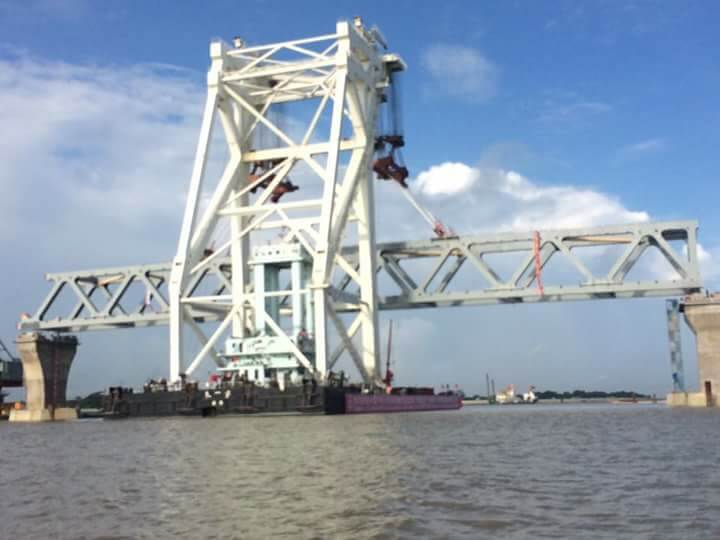July 5, 2022

## How to convert mg/l to meq/l:

In hydrological research, most of the time we need to convert the mg/l to meq/l. This milligram per litre is also a unit. The milliequivalent per litre is also a unit to measure the cation or anion concentration.

To know the milliequivalent we need to know, what is milliequivalent? This is the atomic mass divided by the valency.

suppose, Ca++ has a valency 2+

Ca++ atomic mass is 40.08 gm/mole

So milliequivalent is the 40.08(gm/mole)/2 (valency/mole)= 20.04 gm/equivalent

Now,  33 mg/L Ca++ need to convert to the milliequivalent/L

is simply devide by 20.04

Hence, 33/ 20.04 meq/L is the answer.#### You may have missed#### NST Fellowship to MS / MPhil, PhD and Post-Doctoral level students/researchers in science and technology related subjects at various universities for the development of science and technology